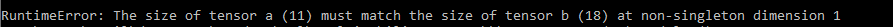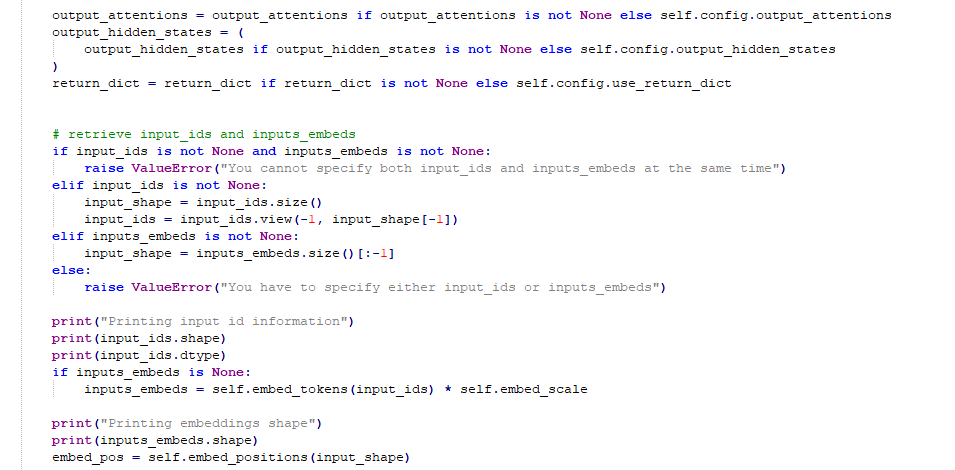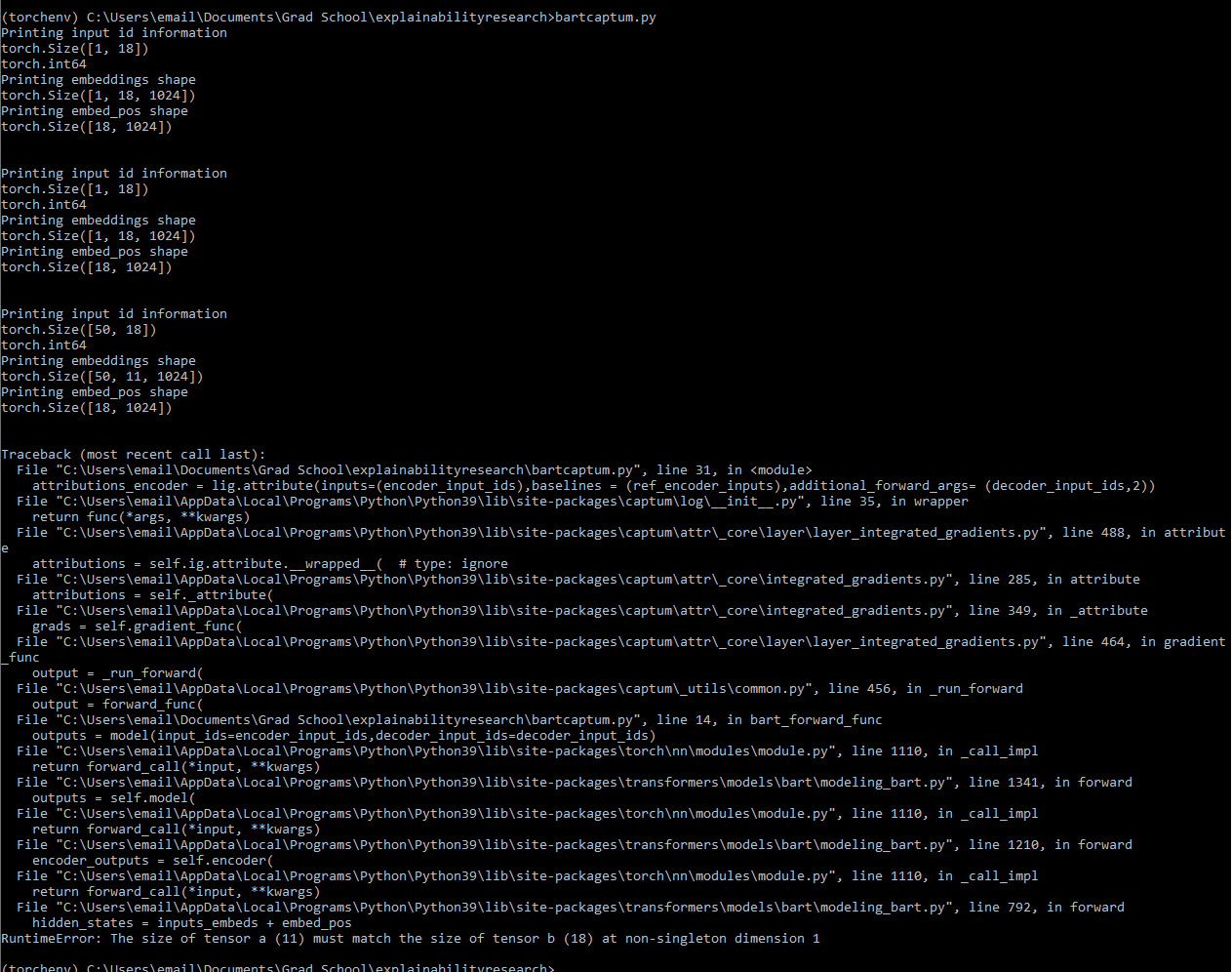# LayerIntegratedGradients throwing error when using BART

I was trying to adapt the BERT tutorial to work with BART. However, when I run the attribution, the forward function eventually breaks. After stepping through the code, I see that LayerIntegratedGradients runs the forward function I specify a couple times before it breaks. First, it runs it with the passed in encoder and decoder input ids (encoder inputs shape: [1,18], decoder inputs shape: [1,11]) and this works fine. Then it runs it with the baseline inputs (which have the same shapes as above), this also works fine. However, the third time, it runs it with some repeated version of my input_ids ( encoder inputs shape: [50,18], decoder inputs shape: [50,11]). This time, the forward function breaks when trying to run through the BART model and gives me the error:I traced through the Bart code to see why this was happening and traced it down to the beginning of the BartEncoder forward function in modelling_bart.py when it turns the input_ids into embeddings and then adds the positional embedding. What is breaking the code is that for some reason when I pass in the encoder id inputs (shape [50,18]) to the embedding layer, instead of giving me [50,18,1024] it gives me [50,11,1024], which is the same length as the decoder input shape! Furthermore, I have verified that the encoder id inputs are actually of shape [50,18] when they are put into the embedding layer. Below I show the code that is causing the break (I have added some print statements in so it is not an exact match with what you would find in huggingface documentation) as well as my print statements demonstrating what is happeningAs we can see here, there are three times the print statements I put into the BartEncoder function are triggered - one when the normal inputs are run, one when the baseline is run, and one when the code breaks. The third time we see that even though the shape of the input_ids is [50,18], the output of the embedding layer is [50,11], which is what I would’ve expected if I was passing in the decoder inputs not the encoder inputs. As a result, when in the next line the code tries to add the position embedding to the input embeddings there is a size mismatch and the code fails. Essentially, the embedding layer is truncating the encoder input length to the decoder sequence length. I am quite confused as to why this is happening and would appreciate help in fixing it. I know the captum code changes up the model a fair bit and I was wondering if there is some side effect of that which I need to correct.

I have provided my code below, and it is a self-contained example for ease of use. If you want to set a breakpoint where the code is breaking, step into the first line of bart_forward_func (where the model is called) and the debugger will display to you the path to your modelling_bart.py file. Then, insert a breakpoint into your modelling_bart.py file at line 777, either by manually putting in a pdb.set_trace() above the line or by adding it in while pdb is running.

``````from transformers import BartTokenizer, BartForConditionalGeneration, utils
import pdb
import torch

tokenizer = BartTokenizer.from_pretrained(model_name)
model = BartForConditionalGeneration.from_pretrained(model_name, output_attentions=True)

def bart_forward_func(encoder_input_ids,decoder_input_ids,index):
outputs = model(input_ids=encoder_input_ids,decoder_input_ids=decoder_input_ids)
pred_idx = decoder_input_ids[0,index]
pred = outputs.logits[:,index-1,pred_idx]
return pred

def generate_ref_sequences(input_ids):
ref_input_ids = torch.zeros_like(input_ids)
ref_input_ids[0,0] = tokenizer.bos_token_id